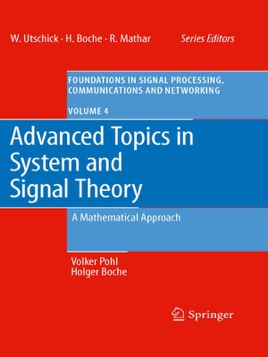• \$159.99

## Publisher Description

This book provides an in-depth analysis of selected methods in signal and system theory with applications to problems in communications, stochastic processes and optimal filter theory. The authors take a consistent functional analysis and operator theoretic approach to linear system theory, using Banach algebra and Hardy space techniques. The themes connecting all the chapters are questions concerning the consequences of the causality constraint, which is necessary in all realizable systems, and the question of robustness of linear systems with respect to errors in the data.

The first part of the book contains basic background on the necessary mathematical tools and provides a basic foundation of signal and system theory. Emphasis is given to the close relation between properties of linear systems such as causality, time-invariance, and robustness on the one hand and the algebraic structures and analytic properties of the mathematical objects, such as Banach algebras or Hardy spaces, on the other hand. The requirement of causality in system theory is inevitably accompanied by the appearance of certain mathematical operations, namely the Riesz projection and the Hilbert transform. These operations are studied in detail in part two. Part three relates the mathematical techniques that are developed in the first two parts to the behaviour of linear systems that are of interest from an engineering perspective, such as expansions of transfer functions in orthonormal bases, the approximation from measured data and the numerical calculation of the Hilbert transform, as well as spectral factorization.